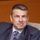cancel
Showing results for
Did you mean:Frequent Visitor

## Iterating over rows based on filter to write multiple if else statement and perform calculationsIn the above table:

1. Need to FILTER  by ([Month Name not equal]="Y"  and based on Asset Number) , since there are many other assets and this has to be done for each asset, ([Month Name not equal]="Y" will filter be Feb March April2. I need to write conditions and then update the next value for New DT

IF[New DT] >= [Hrs Bw St and End Date] then [Hrs Bw St and End Date] else  [New DT] (1779.55>=520.04)  result of new column 520.04

Update [New DT for next month(March)= [New DT]- [Hrs Bw St and End Date] New DT (March)-->1259.51

IF[New DT] >= [Hrs Bw St and End Date] then [Hrs Bw St and End Date] else  [New DT] (1259.51>=744)  result of new column 744

[New DT for next month(April) = [New DT] - [Hrs Bw St and End Date] (April)--> 515.51

IF[New DT] >= [Hrs Bw St and End Date] then [Hrs Bw St and End Date] else  [New DT] (515.51<=516.5) result of new colum 515.51

 Month New column for DT Feb 520.04 March 744 April 515.51

1 ACCEPTED SOLUTIONCommunity Champion

@ASI80486 create this calculated column:

``````Final Column =
VAR _asset = 'asset_downtime (3)'[Asset Number]
VAR _time = 'asset_downtime (3)'[Start Date]
VAR _total = SUMX(FILTER('asset_downtime (3)', 'asset_downtime (3)'[Asset Number] = _asset && 'asset_downtime (3)'[Month Name not equal] = "Y"),'asset_downtime (3)'[New DT])
VAR _substract =
SUMX(
FILTER(
'asset_downtime (3)',
'asset_downtime (3)'[Asset Number] = _asset
&& 'asset_downtime (3)'[Month Name not equal] = "Y"
&& 'asset_downtime (3)'[Start Date] < _time
),
'asset_downtime (3)'[Hrs Bw St and End Date]
)
VAR _result =
SWITCH(
TRUE(),
'asset_downtime (3)'[Month Name not equal] = "N", 'asset_downtime (3)'[New DT],
'asset_downtime (3)'[Hrs Bw St and End Date] <= 'asset_downtime (3)'[New DT], 'asset_downtime (3)'[Hrs Bw St and End Date],
_total - _substract > 'asset_downtime (3)'[Hrs Bw St and End Date], 'asset_downtime (3)'[Hrs Bw St and End Date],
_total - _substract
)
RETURN
MAX(_result, 0 )``````6 REPLIES 6Community Champion

@ASI80486 create this calculated column:

``````Final Column =
VAR _asset = 'asset_downtime (3)'[Asset Number]
VAR _time = 'asset_downtime (3)'[Start Date]
VAR _total = SUMX(FILTER('asset_downtime (3)', 'asset_downtime (3)'[Asset Number] = _asset && 'asset_downtime (3)'[Month Name not equal] = "Y"),'asset_downtime (3)'[New DT])
VAR _substract =
SUMX(
FILTER(
'asset_downtime (3)',
'asset_downtime (3)'[Asset Number] = _asset
&& 'asset_downtime (3)'[Month Name not equal] = "Y"
&& 'asset_downtime (3)'[Start Date] < _time
),
'asset_downtime (3)'[Hrs Bw St and End Date]
)
VAR _result =
SWITCH(
TRUE(),
'asset_downtime (3)'[Month Name not equal] = "N", 'asset_downtime (3)'[New DT],
'asset_downtime (3)'[Hrs Bw St and End Date] <= 'asset_downtime (3)'[New DT], 'asset_downtime (3)'[Hrs Bw St and End Date],
_total - _substract > 'asset_downtime (3)'[Hrs Bw St and End Date], 'asset_downtime (3)'[Hrs Bw St and End Date],
_total - _substract
)
RETURN
MAX(_result, 0 )``````Frequent Visitor

Thank you @SpartaBIfor your quick response and solution. You helped me solve this issue.Thanks so much for your time.Community Champion

@ASI80486 my pleasure 🙂Super User

In Power Query, go to Add Column > Add conditional column. There you'll have a user interface that produces a series of if (else) statements. Start from there, and if you can't do exactly what you want from the UI, you can always tweak the generated code manually from the Advanced Editor.

------------------------------------------------
2. Learning how to fish > being spoon-fed without active thinking.
------------------------------------------------
BI Blog: Datamarts | RLS/OLS | Dev Tools | Languages | Aggregations | XMLA/APIs | Field Parameters | Custom VisualsFrequent Visitor

here the issue is to update the New DT column with new value and then use that updated new DT value in the next IF statement to calculate the next value in the new columnSuper User

Oh, I see, it's on a row-by-row basis. I don't think there's a solution using purely the Power Query UI, I think you'd have to add an index column and write some M code. It's probably something I'd solve in DAX though. Sorry I don't have time to flesh it out further, maybe other people will chime in.

------------------------------------------------
2. Learning how to fish > being spoon-fed without active thinking.
------------------------------------------------
BI Blog: Datamarts | RLS/OLS | Dev Tools | Languages | Aggregations | XMLA/APIs | Field Parameters | Custom Visuals